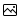Learning never exhausts the mind
Home >  Astronomy > Learn Astronomy > What Are Lagrange Points?

# What Are Lagrange Points?

First Published 9th February 2011, Last Updated by

Lagrange Points are five special points where an object can orbit at a fixed distance from two larger mass such that the gravitational pull precisely equals the centripetal force.
Introduction to Astronomy Series

The Lagrange Points were discovered by the Italian-French mathematician Joseph-Louis Lagrange.

There are five Lagrange points to be found in the vicinity of two orbiting masses. Of the five Lagrange points, three are unstable and two are stable. The unstable Lagrange points - labelled L1, L2 and L3 - lie along the line connecting the two large masses. The stable Lagrange points - labelled L4 and L5 - form the apex of two equilateral triangles that have the large masses at their vertices.

Lagrange points are analogous to geostationary orbits in that they allow an object to be in a fixed position in space rather than an orbit in which its relative position changes continuously.

Lagrange points are the stationary solutions of the circular restricted three-body problem. For example, given two massive bodies in circular orbits around their common centre of mass, there are five positions in space where a third body, of comparatively negligible mass, could be placed which would then maintain its position relative to the two massive bodies. As seen in a rotating reference frame with the same period as the two co-orbiting bodies, the gravitational fields of two massive bodies combined with the satellite's circular motion are in balance at the Lagrangian points, allowing the third body to be stationary with respect to the first two bodies.

The first three Lagrange points are nominally unstable. While it is possible to find stable periodic orbits around these points, if an object located at the L1 point drifted closer to one of the masses, the gravitational attraction it felt from that mass would be greater, and it would be pulled closer. The pattern is very similar to that of tidal forces.

In contrast to the unstable Lagrangian points, the triangular points (L4 and L5) are stable, provided that the ratio of M1/M2 is greater than 24.96. This is the case for the Sun-Earth system, the Sun-Jupiter system, and, by a smaller margin, the Earth-Moon system. When a body at these points is perturbed, it moves away from the point, but the factor opposite of that which is increased or decreased by the perturbation (either gravity or angular momentum-induced speed) will also increase or decrease, bending the object's path into a stable, kidney bean?shaped orbit around the point as shown in the contour lines in the image below.

L4 and L5 are sometimes called triangular Lagrange points or Trojan points. The name Trojan points comes from the Trojan asteroids at the Sun-Jupiter L4 and L5 points, which themselves are named after characters from Homer's Iliad (the legendary siege of Troy). Asteroids at the L4 point, which leads Jupiter, are referred to as the "Greek camp", while at the L5 point they are referred to as the "Trojan camp". These asteroids are (largely) named after characters from the respective sides of the Trojan War.Trojan asteroids of Jupiter (coloured green) in front of (the "Greeks") and behind Jupiter along its orbital path. Also shown is the main asteroid belt between the orbits of Mars and Jupiter (white), and the Hilda family of asteroids (brown).

Wikipedia

## Lagrangian Point Missions

The Lagrangian point orbits have unique characteristics that have made them a good choice for performing some kinds of missions. These missions generally orbit the points rather than occupy them directly.

MissionLagrangian pointAgencyStatus